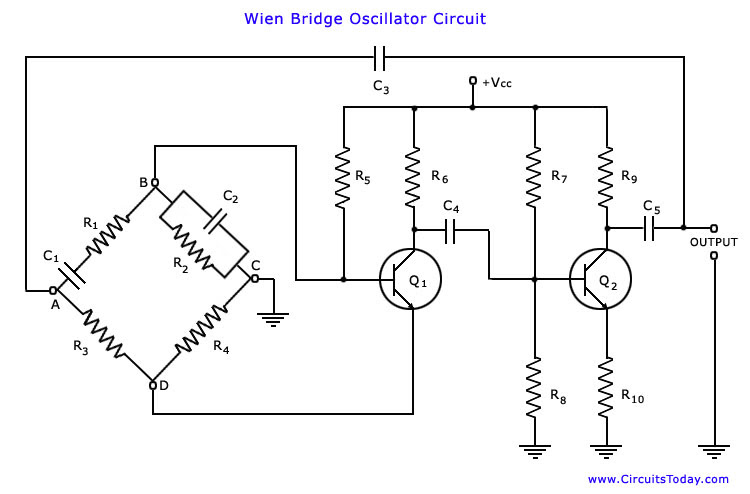## Audio

[Audio][slideshow]

It is one of the most popular type of oscillators used in audio and sub-audio frequency ranges (20 – 20 kHz). This type of oscillator is simple in design, compact in size, and remarkably stable in its frequency output. Furthermore, its output is relatively free from distortion and its fre­quency can be varied easily. However, the maximum frequency output of a typical Wien bridge oscillator is only about 1 MHz. This is also, in fact, a phase-shift oscillator. It employs two transistors, each producing a phase shift of 180°, and thus producing a total phase-shift of 360° or 0°.

The circuit diagram of Wien bridge oscillator is shown in the figure below.It is essentially a two-stage amplifier with an R-C bridge circuit. R-C bridge circuit (Wien bridge) is a lead-lag network. The phase’-shift across the network lags with increasing frequency and leads with decreasing frequency. By adding Wien-bridge feedback network, the oscillator becomes sensitive to a signal of only one particular frequency. This particular frequency is that at which Wien bridge is balanced and for which the phase shift is 0°.If the Wien-bridge feedback network is not employed and output of transistor Q2 is fedback to transistor Q1 for providing regeneration re­quired for producing oscillations, the transistor Q1 will amplify signals over a wide range of frequencies and thus direct coupling would result in poor frequency stability. Thus by em­ploying Wien-bridge feedback network frequency stability is increased.

In the bridge circuit R1 in series with C1, R3, R4 and R2 in parallel with C2 form the four arms.

This bridge circuit can be used as feedback network for an oscillator, provided that the phase shift through the amplifier is zero. This requisite condition is achieved by using a two stage amplifier, as illustrated in the figure. In this arrangement the output of the second stage is supplied back to the feedback network and the voltage across the parallel combination C2 R2 is fed to the input of the first stage. Transistor Q1 serves as an oscillator and amplifier whereas the transistor Q2 as an inverter to cause a phase shift of 180°. The circuit uses positive and negative feedbacks. The positive feedback is through R1 C1 R2, C2 to tran­sistor Q1 and negative feedback is through the voltage divider to the input of transistor Q1. Resistors R3 and R4 are used to stabilize the amplitude of the output.

The two transistors Q1 and Q2 thus cause a total phase shift of 360° and ensure proper positive feedback. The negative feedback is provided in the circuit to ensure constant output over a range of frequencies. This is achieved by taking resistor R4 in the form of a tempera­ture sensitive lamp, whose resistance increases with the increase in current. In case the amplitude of the output tends to increase, more current would provide more negative feedback. Thus the output would regain its original value. A reverse action would take place in case the out­put tends to fall.

The amplifier voltage gain, A R3 + R4 / R4 = R3 / R4 + 1 = 3

Since R3 = 2 R4

The above corresponds with the feedback network attenuation of 1/3. Thus, in this case, voltage gain A, must be equal to or greater than 3, to sustain oscillations.

To have a voltage gain of 3 is not difficult. On the other hand, to have a gain as low as 3 may be difficult. For this reason also negative feedback is essential.

### Operation

The circuit is set in oscillation by any random change in base current of transistor Q1, that may be due to noise inherent in the transistor or variation in voltage of dc supply. This variation in base current is amplified in collector circuit of transistor Q1 but with a phase-shift of 180°. the output of transistor Q1 is fed to the base of second transistor Q2 through capacitor C4. Now a still further amplified and twice phase-reversed signal appears at the collector of the transistor Q2. Having been inverted twice, the output signal will be in phase with the signal input to the base of transistor Q1 A part of the output signal at transistor Q2 is fedback to the input points of the bridge circuit (point A-C). A part of this feedback signal is applied to emitter resistor R4 where it produces degenerative effect (or negative feedback). Similarly, a part of the feedback signal is applied across the base-bias resistor R2 where it produces regenerative effect (or positive feedback). At the rated frequency, effect of regeneration is made slightly more than that of degeneration so as to obtain sustained os­cillations.

The continuous frequency variation in this oscillator can be had by varying the two capacitors C1 and C2 simultaneously. These capacitors are variable air-gang capacitors. We can change the frequency range of the oscillator by switching into the circuit different values of resistors R1 and R2.